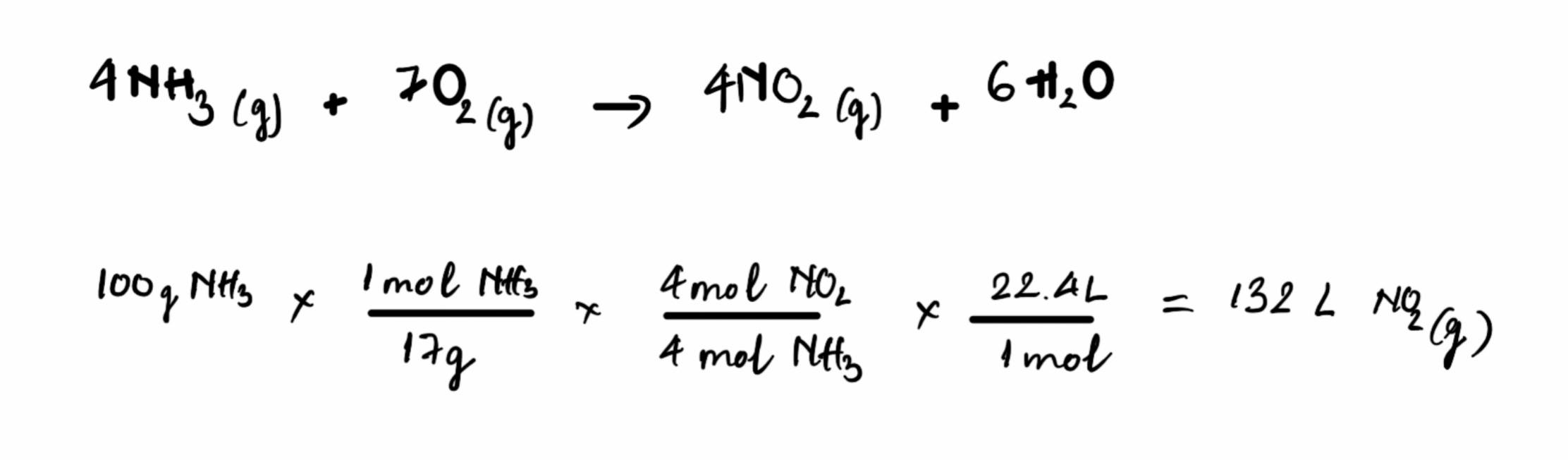MCAT Content / Gas Phase / Molar Volume At 0c And 1 Atm 22 4 L Mol 2

### Molar Volume at 0°C and 1 atm = 22.4 L/mol

Topic: Gas Phase

At standard temperature and pressure, one mole of any gas will occupy a volume of 22.4 L.

Stoichiometry is the quantitative study of the relative amounts of reactants and products in chemical reactions; gas stoichiometry involves chemical reactions that produce gases. Stoichiometry is based on the law of conservation of mass, meaning that the mass of the reactants must be equal to the mass of the products. This assumption can be used to solve for unknown quantities of reactants or products.

Stoichiometric calculations involving gases allow us to convert between mass, number of moles, and most importantly, volume of gases. The following relationship makes this possible:

1 mole of any gas at standard temperature and pressure (273 K and 1 atm) occupies a volume of 22.4 L.

While the above relationship is an estimation, it is a relatively good approximation at STP, and can be used reliably in calculations.

For example:Practice Questions

Chlorofluorocarbons and the environment

MCAT Official Prep (AAMC)

Chemistry Question Pack Question 54

Chemistry Question Pack Passage 15 Question 83

Key Points

• At Standard Temperature and Pressure (STP), 1 mole of any gas will occupy a volume of 22.4 L.

• The Ideal Gas Law, along with a balanced chemical equation, can be used to solve for the amount, either in volume or mass, of gas consumed or produced in a chemical reaction.

Key Terms

Standard Temperature and Pressure (STP): 273 K and 1 atm

stoichiometry: the study and calculation of quantitative (measurable) relationships of the reactants and products in chemical reactions (chemical equations)

Billing Information